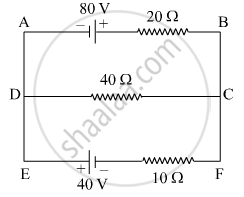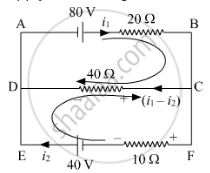# Solve the Following Question. Using Kirchhoff’S Rules, Calculate the Current Through the 40 ω and 20 ω Resistors in the Following Circuit. - Physics

Numerical

Solve the following question.
Using Kirchhoff’s rules, calculate the current through the 40 Ω and 20 Ω  resistors in the following circuit.#### Solution

Apply KVL through ABCDA80 - 20i_1 - 40(i_1 - i_2) = 0

80 - 60i_1 + 40i_2 = 0    ....(1)

Apply KVL through FEDCF

40 + 40(i_1 - i_2) - 10i_2 = 0

40 + 40i_1 - 50i_2 = 0    ......(2)

4i_2 - 6i_1 = -8 (1)       .....[ from (1)]

-5i_2 + 4i_1 = -4 (2)    .....[ from (2)]

Multiply equation (2) by 6/4 and add with equation (1)

4i_2 - 6i_1 = -8

(-30i_2)/4 + 6i_1 = -6
__________________________

4i_2 - 30/4i_2 = -14

(-14)/4i_2 = -14

i_2 = (-14)/7 xx 2

i_2 = 4 A

Put the value of i2 in equation (1)

4 xx (4) - 6i_1 = -8

16 - 6i_1 = -8

6i_1 = 16 + 8 = 24

i_1 = 4A

So, current through 40 Ω resistor = i_1 - i_2

= 4 - 4

= 0 A

Hence, current through 20 Ω resistor = 4A.

Concept: Kirchhoff’s Rules
Is there an error in this question or solution?